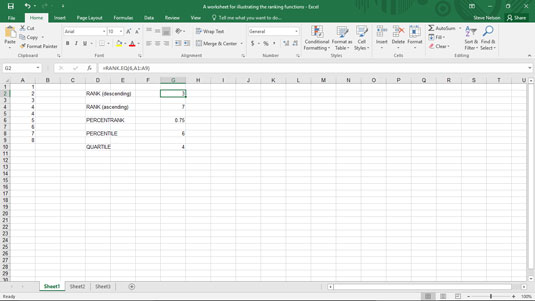##### Excel Data Analysis For DummiesExcel provides functions for finding values with a particular rank and for ranking values within the data set. The RANK functions determine the rank, or position, of a value in an array. All the RANK functions use the syntax

```=RANK(number,ref,[order])
=RANK.AVG(number,ref,[order])
=RANK.EQ(number,ref,[order])```

where number is the value you want to rank, ref is the array of values, and optionally order indicates whether array values should be arranged in descending order (indicated with a 0 or logical FALSE value) or in ascending order (indicated with a 1 or logical TRUE value). By the way, Excel ranks duplicate values the same, but these duplicates do affect the rank of subsequent numbers. If you leave out the order argument, Excel ranks values in descending order.

To demonstrate how the RANK function works, suppose you want to rank the values shown in the worksheet range A1:A9 in this figure.A worksheet fragment with the array 1, 2, 3, 4, 4, 5, 6, 7, 8.

The formula in cell G2

`=RANK(6,A1:A9)`

returns the value 3, indicating that when a descending order is used, the value 6 is the third value in the array.

The formula in cell G4

`=RANK(6,A1:A9,1)`

returns the value 7, indicating that when an ascending order is used, the value 6 is the seventh value in the array.

Note that the RANK.EQ function returns the same value as the RANK function. The RANK.AVG function, however, calculates the average rank of a value when you have duplicate values in an array.

For example, both RANK(6,A1:A9,1) and RANK.EQ(6,A1:A9,1) return 2 if the array holds the values 1,2,3,4,4,5,6,6,8, because 2 is the second value in the array when you arrange values in descending order. However, if you use the formula RANK.AVG(6,A1:A9) to find the rank of the value 6 in that array, Excel returns 2.5 because the value 6 appears both in the number 2 and in the number 3 spot, so its average rank is 2.5.